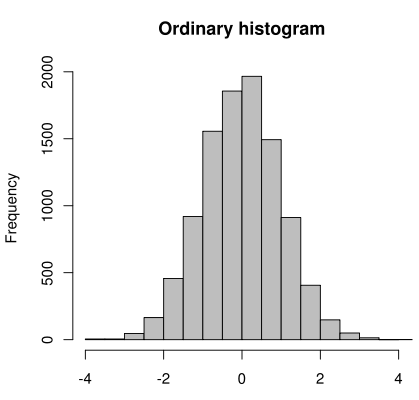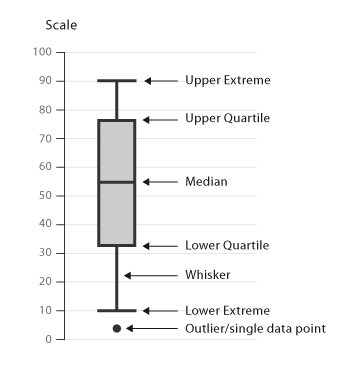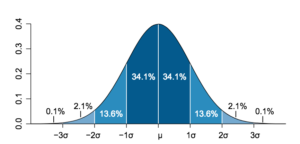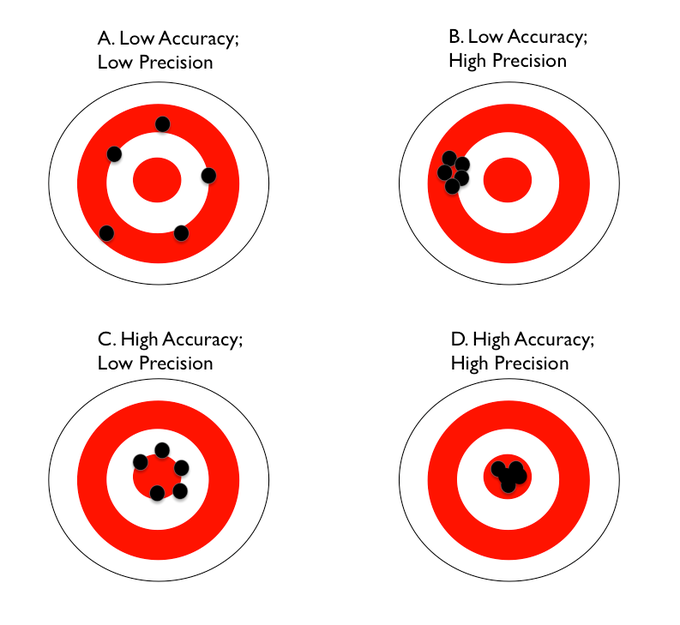## Basic Descriptive Statistics

The goal of statistics is to summarize data in a manner that allows for easy descriptions or inferences to be made.

### Learning Objectives

Explain the descriptive statistics used to measure central tendency and variability

### Key Takeaways

#### Key Points

• The mean is the average value of a data set. In the data set (1, 3, 3, 4, 5, 6), the average would be the sum of all of the values of the data set (1+3+3+4+5+6 = 22), divided by the total number of values (6), which gives a mean of 3.66 for this data set.
• The median is the value found in the middle of an ordered set of data. In the data set (1, 3, 3, 4, 5, 6), which is ordered from smallest to largest, the median would be the value found between the two middle numbers of the set 3 and 4. Thus the median of this data set is 3.5.
• The mode is the most common value in a data set. In the data set (1, 3, 3, 4, 5, 6), 3 is the mode because it is the value that appears the most number of times.
• The range is the difference between the highest and lowest values of a data set, generally found by subtracting the lowest from the highest value found. In the data set (1, 3, 3, 4, 5, 6), the range is 6-1 = 5.
• The variance is the mean squared deviation of a data set, meaning it is the sum of the squared deviations of the mean divided by the total population.
• The standard deviation is calculated as the square root of the variance. This resulting quantity is in the same units as the original values, which overcomes certain limitations for interpreting the variance.

#### Key Terms

• standard deviation: A measure of how spread out data values are around the mean, mathematically defined as the square root of the variance.
• ratio: A number representing a comparison of two quantities, usually represented as a fraction, percentage, or decimal.
• ordinal: The position at which a number appears in a sequence, such as first, second, or third.

Statistics deals with the collection, analysis, interpretation, and presentation of numerical data. Once data are gathered from an experimental study, it must be put into some kind of form—usually numerical—to be analyzed. The goal of statistics is to summarize data in a manner that allows for easy descriptions or inferences to be made.

Descriptive statistics involves two major aspects of data: central tendency and variance. Central tendency and variability measures are used to interpret the meaning and value of data. Once these measures have been determined, graphs and charts are often used to illustrate the results of the data in a clear and concise manner.

### Central Tendency

Central tendency is most commonly referred to as the numerical center of the data set: it is a single number that is used to represent a group of numbers. There are three common representations of central tendency: the mean, median, and mode.

The mean is the average value of a data set. In the data set (1, 3, 3, 4, 5, 6), the average would be the sum of all of the values of the data set (22), divided by the total number of values (6), which gives a mean of 3.66 for this data set. The mean can only be calculated with interval and ratio data. One limitation of the mean is that it is significantly influenced by outliers (numbers which fall far from the data set’s median); when this happens, the median becomes a better measure of the data set’s center.

The median is the value found in the middle of an ordered set of data (where the data set is ranked from lowest to highest). If the data set is made up of an even number of values, then the two middle values should be averaged to find the median. In the data set (1, 3, 3, 4, 5, 6), the median would be the value found between the two middle numbers of the set 3 and 4. Thus the median of this data set is 3.5. The median can be computed for ordinal, interval, and ratio data.

The mode refers to the most commonly occurring value in a data set. In the data set (1, 3, 3, 4, 5, 6), 3 is the mode because it is the value which appears most often; because it is the only mode, it is considered to be the single modal value. In a similar data set (1, 3, 3, 4, 5, 5, 6), the mode would be both the values 3 and 5; this would be a multi-modal value. The mode is the least influential measure of central tendency because little insight is gathered from its calculation.

### Variability Measures

A data set involves a range of values. The standard deviation and variance are related and involve how much individual data differs from the data set’s mean. There are three variability measures of a data set: range, standard deviation, and variance.

The range is the difference between the highest and lowest values of a variable, often found by subtracting the lowest from the highest value found. The range is often an unstable identifier of variability because it can be overly influenced by a single outlying value, and often these outliers are removed from the data set to ensure more representative results.

The variance is the mean squared deviation of a data set, meaning it is the sum of the squared deviations of the mean divided by the total population. It is the sum of the deviation squared divided by the number of values—thus, the average distance of a value from the mean.

$\displaystyle{\frac{\Sigma{(x-\bar{x})^2}}{N}}$

The standard deviation is calculated as the square root of the variance. This resulting quantity is in the same units as the original values, overcoming the limitation on interpreting the variance.

$\displaystyle{\sqrt{\frac{\Sigma{(x-\bar{x})^2}}{N}}}$

Another way to measure the variability of the data is through skewness. Ideally, a variable will be normally distributed, meaning that the mean, median, and mode are all identical. If the mean is greater than the median, and the median is greater than the mode, the distribution will be positively skewed. However, if the mean is less than the median, and the median is less than the mode, then the distribution will be negatively skewed.Skewness:  When the mean, median, and mode are unequal, the normal curve can become skewed in either a negative or positive direction depending on their values in relation to each other. In each of the above panels, the dotted line shows how a normal distribution would be shaped (if the mean and median were equal). In the left panel, showing negative skew, the mean is less than the median; in the right panel, showing positive skew, the mean is greater than the median.

### Visualizing Descriptive Statistics

When a researcher computes descriptive statistics, they will usually want to create visual representations to help them better understand their data. This is better known as “exploratory data analysis”. Often researchers will want to start with a frequency distribution, which is simply a table that organizes the data in a logical order so they can count the number of times each value occurred. For example, if we have collected reaction times of people responding to a stimulus, a frequency distribution would allow us to see the number of times each possible reaction time occurred.

### Stem-and-Leaf Plot

A stem-and-leaf plot is one way to visualize the frequencies. Stem-and-leaf plots retain the individual values and the frequencies of those values, and also give an idea of the shape of the distribution of the data.Stem-and-leaf plot: “Leading numbers” are shown in the “stem” (the column on the left-hand side), and “trailing numbers” are shown in the “leaves” (the lists on the right-hand side). In this example, the first row represents the numbers 1, 1, 2, 2, etc.; the second row represents the numbers 10, 10, 10, 11, 11, 13, etc.; and so on.

### Histogram

A histogram helps researchers visualize the distribution of their data. Each histogram contains columns that are used to represent the number of data points within each interval, or “bin.”Histogram: This histogram displays normally distributed data (it is shaped like a symmetrical bell, meaning the median is roughly equal to the mean). Each column represents the number of data points of observations within that interval, or “bin.” For example, the first two intervals, or “bins,” are on the x-axis, and frequencies are on the y-axis.

### Boxplot

A researcher may also want to use a boxplot to better understand the variability in a sample. A boxplot is a graphical representation of the dispersion of data. A boxplot provides information about the median value, the spread of the data and range, and statistical outliers.Boxplot: A boxplot has marks to indicate the upper and lower extreme, as well as the upper and lower quartile and median of the data.

## Basic Inferential Statistics

Statistical inference makes claims about a population of individuals or things, using data drawn from a smaller subset of the population known as a sample.

### Learning Objectives

Analyze the components of a normally distributed population

### Key Takeaways

#### Key Points

• Because it is usually impossible to study an entire population, researchers will collect data from a smaller subset, known as a sample, of that population. Statistical inference then allows the researcher to draw conclusions about the larger population based on the sample.
• Researchers will want to ensure that their sample is truly random, meaning every characteristic of the population of interest has an equal chance of being included in the sample.
• If a data set is normally distributed, that means the mean, median, and mode of that data set are all approximately equal. The curve is bell-shaped, and 68% of the values lie within one standard deviation of the mean, and 96% within two standard deviations.
• Most inferential statistics are based on the assumption that the variable we are measuring is normally distributed.

#### Key Terms

• parameter: A numerical value that gives information about an entire population, where a population is a complete group of individuals or things.
• population: A complete group of individuals or things that research aims to describe.
• sample: A smaller subset of a population of individuals or things.
• random sample: A smaller subset of a population of individuals or things in which every characteristic present in the population has an equal chance of being represented.
• statistics: Numerical values that give information about a sample of individuals or things, where the sample is a smaller subset of a population of interest.

When psychologists want to test a research hypothesis, they will usually need to use statistical inference. Statistical inference makes propositions about a population by using a sample, which is data drawn from that population.

### Understanding Populations and Samples

Suppose we are interested in studying whether there is a difference between the annual income of women over 30 who have a college education as compared with those who do not. A “population” includes the complete set of people (or items) with the characteristic we wish to understand. In this case, our population is all women over 30. However, because it would be very difficult (and very costly in terms of time and money) to gather information from every woman over 30, we need to take a representative sample of the population, and use that sample to infer something about the characteristics of the entire population. A “sample” is a set of observations derived from a subset of a population.

In our example above, it would be very difficult to collect information on the entire population (women over 30), but in some cases, getting information on the entire population would be impossible. Such populations include, for example, the length of all supermarket checkout lines, the height of every person in the world, or the age at which every child on Earth first began to walk. Because of this, it is important to note that a population can range from a relatively small, specific set of numbers (all female students at Harvard University) to an infinitely large set of numbers which could never be collected (the height of every person in the world). This is the practical reason why that we must collect samples.

### Statistics and Parameters

When we collect our sample, we compute numerical values that summarize the data in our sample. For example, when we collect a sample of women over 30, we might collect their mean income and mean education level. These values are called statistics. The corresponding values in the population are called parameters. Statistical inference therefore literally helps us make inferences about the characteristics of populations (their parameters) from characteristics of our sample (statistics). Because it is typically impossible to study an entire population, a sample gets us as close as possible, and statistical inference enables us to infer the characteristics of our population.

### Sample Representation

Because a sample is the key to better understanding characteristics of the population we are interested in, a researcher must put considerable thought into how he or she will sample from that population. Researchers will ideally want to strive to ensure that their sample is truly random. In a “ random sample,” every element of the population has an equal chance of being included in the sample. Collecting a true random sample helps the researcher ensure that the statistics they are using to make inferences about a population are accurate. For example, to collect a random sample of women over 30 for our study above, we may get a census list of women over 30 in the population and send a survey about income to every 10th woman on the list.

Although random samples are the ideal, researchers will often end up using samples of convenience instead (e.g., volunteers from an Introduction to Psychology class) because truly random samples are difficult to obtain and often impractical. Although convenience samples are not as ideal as a random sample, they are easy to collect, and depending on the research question, may provide an good enough approximation of the population being studied.

It is also important to note that sample size is a crucial factor to take into account when conducting a study. The bigger the sample the researcher collects, the closer the researcher gets to getting more accurate estimates of population parameters. Accordingly, depending on several other factors, larger samples can also give the researcher a greater chance that their results will be statistically significant, meaning that it can increase their power to detect an effect.

### The Normal Distribution

For a distribution that is perfectly normally distributed, the mean is equal to the median, as well as the mode. The normal distribution is expressed in terms of standard deviation around the mean, where 68% of values lie within one standard deviation of the mean, and where approximately 96% of the values lie within two standard deviations of the mean.Normal distribution: The normal, or Gaussian, distribution of values (also known as the bell curve). This occurs when the mean, median, and mode are equivalent, or approximately equivalent.

The reason the normal distribution is so important is because most inferential statistics are based on the assumption that the variable we are measuring is normally distributed. If our variable is normally distributed, that gives us confidence that if we were to obtain the whole population of observations for that variable, the resulting distribution would also be normally distributed, and therefore the inferences we draw (using statistics) are accurate.

### Inferential Statistical Tests

What type of inferential statistics we use will inevitably depend on our research question and our type of data. There are many types of inferential statistical tests, but perhaps the simplest is the t-test, which determines whether there is a significant difference between two means. For example, we may want to compare SAT scores between high school senior women and men to see if there is a difference. We would first collect our sample from the population, ideally a random one. Next, we would collect their SAT scores and compute the mean SAT score for women and the mean SAT score for men. Then we would run an independent samples t-test, which would tell us whether there is a significant difference between mean SAT scores for men versus women.

However, there are many other types of inferential statistics that can be used, some of which test differences in means (t-tests), others which test differences in variances (analysis of variance), and others which use more sophisticated statistical models (General Linear Models). It is up to the researcher to decide which statistical test is the correct fit for their data.

## Accuracy vs. Precision

In order to be valid, data must be both accurate and precise.

### Learning Objectives

Discuss why data must be both accurate and precise in order to be valid

### Key Takeaways

#### Key Points

• When researchers collect data, they strive for both accuracy and precision so that their data are valid.
• Accuracy is the closeness of a measurement to the quantity’s true value—i.e., the “correctness” of the data.
• Precision is the degree to which two or more repeated measurements show the same results each time—i.e., the reliability or consistency of the data.
• Measurement error can be either systematic (i.e., the same error applied to every measurement) or random (i.e., the mistake occurred with only a few measurements).

#### Key Terms

• Accuracy: The closeness of a measurement of a quantity to that quantity’s true value.
• Precision: The degree to which multiple measurements of the same thing show the same or similar results each time.

### Understanding Accuracy vs. Precision

When scientists conduct research, they strive to collect data that is both accurate and precise. If their data is both accurate and precise, this gives them confidence that it is valid.

### Accuracy

“Accuracy” is the closeness of a measurement quantity to that quantity’s true value. In other words, it is the extent to which the measurement is error free.  For example, if Dr. Smith is collecting data on people’s height and she measures someone who is rather short—63 inches (5’2″ and a half)—at exactly 63.0 inches, she is accurate. However, if Dr. Smith collects a measurement for that person that is 67.5 inches, her measurement is now inaccurate because it is no longer close to the true value.

### Precision

“Precision” is the degree to which two or more repeated measurements show the same results each time. Sometimes precision is referred to as the reliability or consistency of the measurement.

Let’s return to our example above: Dr. Smith, who is collecting data on height. Often researchers will take repeated measurements of the same entity for a variety of reasons related to data quality. Dr. Smith is collecting data on each person’s height three times for her study. If she measures that same 63-inch tall person three times, and collects measurements of 67.5, 67.4, and 67.4, this means that she has collected very precise measurements. Note that her measurements are not accurate, however: they are far from this person’s actual height of 63 inches. In this way, measurements can be quite precise, but not accurate. Thankfully Dr. Smith’s grad student also measures the same 63-inch tall person and obtains measurements of 63.0, 63.01, and 63.0. The grad student’s measurements are not only precise, but accurate as well because they are consistent and also very close to the true height.

Note that measurements can also be accurate, but not precise. In the above example, if Dr. Smith obtained measurements of 63, 64, and 62.5, these would be accurate because they are close to the person’s true height, but not precise, because they lack consistency.Accuracy vs. precision: Accurate hits fall close to the bullseye, as shown in dart boards C and D. Precise hits are characterized by the close clustering of consistent hits, shown in dart boards B and D.

Let’s look at another example. If a basketball player is taking shots, she is accurate if her aim always takes the ball close to, or into, the basket. If her aim takes the ball to the same location each time, whether or not it’s close to the basket, she is precise. A great basketball player is accurate and precise by shooting the ball exactly the same way each time and getting it into the basket.

### Measurement Error

When researchers collect data, they strive for both accuracy and precision so that their data are valid and they can reduce variance in their results due to error. Measurement error can occur for a variety of reasons. Suppose you are measuring the weight of various objects in your physics class, but your scale is off by.5 oz. This small difference introduces systematic error into your measurements. The errors would be “systematic”  because the same amount of error would be introduced each time you weighed something. However, if you and your lab mate simply overestimate or underestimate the weight each time because you are not paying attention, this would be an example of “random” error. The error is random because it will vary each time due to human error.